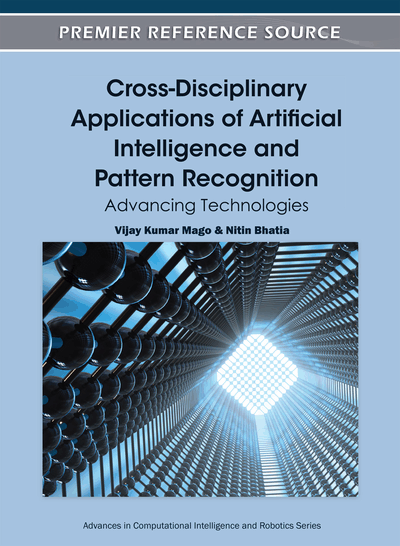# A New Optimization Approach to Clustering Fuzzy Data for Type-2 Fuzzy System Modeling

Mohammad Hossein Fazel Zarandi (Amirkabir University of Technology, Iran) and Milad Avazbeigi (European Centre for Soft Computing, Spain)
DOI: 10.4018/978-1-61350-429-1.ch025
Available
\$37.50
No Current Special Offers

## Abstract

This chapter presents a new optimization method for clustering fuzzy data to generate Type-2 fuzzy system models. For this purpose, first, a new distance measure for calculating the (dis)similarity between fuzzy data is proposed. Then, based on the proposed distance measure, Fuzzy c-Mean (FCM) clustering algorithm is modified. Next, Xie-Beni cluster validity index is modified to be able to valuate Type-2 fuzzy clustering approach. In this index, all operations are fuzzy and the minimization method is fuzzy ranking with Hamming distance. The proposed Type-2 fuzzy clustering method is used for development of indirect approach to Type-2 fuzzy modeling, where the rules are extracted from clustering fuzzy numbers (Zadeh, 1965). Then, the Type-2 fuzzy system is tuned by an inference algorithm for optimization of the main parameters of Type-2 parametric system. In this case, the parameters are: Schweizer and Sklar t-Norm and s-Norm, a-cut of rule-bases, combination of FATI and FITA inference approaches, and Yager parametric defuzzification. Finally, the proposed Type-2 fuzzy system model is applied in prediction of the steel additives in steelmaking process. It is shown that, the proposed Type-2 fuzzy system model is superior in comparison with multiple regressions and Type-1 fuzzy system model, in terms of the minimization the effect of uncertainty in the rule-base fuzzy system models an error reduction.
Chapter Preview
Top

## Introduction

Clustering methods have been used extensively in computer vision and pattern recognition. In recent years, fuzzy clustering methods have gained a lot of attentions because of their advantageous over crisp clustering in that the total commitment of a vector to a given class is not mandatory i.e. an instance could be a member of more than one class at the same time. Also, fuzzy clustering methods have shown their capability to detect not only volume clusters, but also clusters which are actually thin shells i.e. curves and surfaces.

Most analytic fuzzy clustering approaches are derived from the fuzzy C-means (FCM) algorithm (Celikyilmaz & Turksen, 2007). The FCM uses the probabilistic constraint that the membership of a data point across classes sums to 1. The constraint was used to generate the memberships update equations for an iterative algorithm. However, the memberships resulted from FCM and its variations do not always correspond to the intuitive concept of belonging or compatibility. Moreover, these algorithms usually do not produce good results in confront of noisy data. Melek et al. (2005) summarizes the main problems of classic FCM as:

• In order to get the optimal partitions, initial locations of the clusters’ centers should be assigned. Different choices of initial clusters’ centers lead to different clusters and The FCM algorithm always converges to local optima.

• There is no well structure, scientific solution for the choice of the weight exponent (m).

• The number of clusters is an argument of FCM and must be known before running FCM. Actually, this is an optimization problem that should be solved before clustering.

The main goal of this research is to present a systematic fuzzy system modeling based on a modified Fuzzy c-Mean algorithm that tries to address the mentioned problems of the FCM. For this purpose, a new distance measure is defined for measuring the distance between fuzzy points. The procedure of the proposed fuzzy system modeling approach is as follows:

• Feature Selection.

• Implementation of Type-2 fuzzy clustering algorithm for generation of Type-2 fuzzy membership functions (Castillo O. & Melin P. 2008; Wu, D.& Mendel, J. M., 2007;).

• Modification of Xie-Beni (1991) cluster validity index to optimize the weighting exponent of data (mˈ) and the number of clusters (c). In order to choose the cluster validity index, some researches have evaluated many cluster validity indexes and Xie-Beni has shown the best performance among them base on the experimental results (Celikyilmaz and Burhan Turksen, 2008; Wu and Mendel, 2009; Weina and Yunjie, 2007).

• Assignment of the most suitable membership functions of the output variable by using of Neural Networks.

• Projection of the output space onto the input spaces to select the most effective input variables.

• Assignment of the most suitable membership functions of the input variables by using Neural Networks.

• Tuning the system by using inference algorithm.

Top

## Fuzzy Cluster Analysis

In general, the aim of cluster analysis is to partition a given set of data or objects into clusters. The objective of a clustering approach is to minimize the compactness of data within the clusters as well as separation of the clusters. The most popular method of fuzzy clustering is Fuzzy c-Mean (FCM), proposed by Bezdek (1981):

(1)

## Complete Chapter List

Search this Book:
Reset